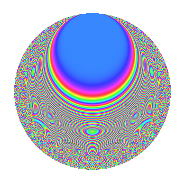# Properties

 Label 861.2.eLevel 861 Weight 2 Character orbit e Rep. character $$\chi_{861}(860,\cdot)$$ Character field $$\Q$$ Dimension 108 Newforms 2 Sturm bound 224 Trace bound 1

# Related objects

## Defining parameters

 Level: $$N$$ = $$861 = 3 \cdot 7 \cdot 41$$ Weight: $$k$$ = $$2$$ Character orbit: $$[\chi]$$ = 861.e (of order $$2$$ and degree $$1$$) Character conductor: $$\operatorname{cond}(\chi)$$ = $$861$$ Character field: $$\Q$$ Newforms: $$2$$ Sturm bound: $$224$$ Trace bound: $$1$$ Distinguishing $$T_p$$: $$2$$

## Dimensions

The following table gives the dimensions of various subspaces of $$M_{2}(861, [\chi])$$.

Total New Old
Modular forms 116 116 0
Cusp forms 108 108 0
Eisenstein series 8 8 0

## Trace form

 $$108q - 112q^{4} + 4q^{9} + O(q^{10})$$ $$108q - 112q^{4} + 4q^{9} + 104q^{16} + 4q^{18} - 16q^{21} + 76q^{25} - 56q^{36} - 16q^{37} - 36q^{39} + 24q^{42} - 16q^{43} - 96q^{46} + 20q^{49} - 12q^{51} - 36q^{57} - 80q^{64} - 14q^{72} - 46q^{78} - 52q^{81} + 30q^{84} - 48q^{91} + O(q^{100})$$

## Decomposition of $$S_{2}^{\mathrm{new}}(861, [\chi])$$ into irreducible Hecke orbits

Label Dim. $$A$$ Field CM Traces $q$-expansion
$$a_2$$ $$a_3$$ $$a_5$$ $$a_7$$
861.2.e.a $$28$$ $$6.875$$ $$\Q(\sqrt{-287})$$ $$0$$ $$0$$ $$0$$ $$0$$
861.2.e.b $$80$$ $$6.875$$ None $$0$$ $$0$$ $$0$$ $$0$$